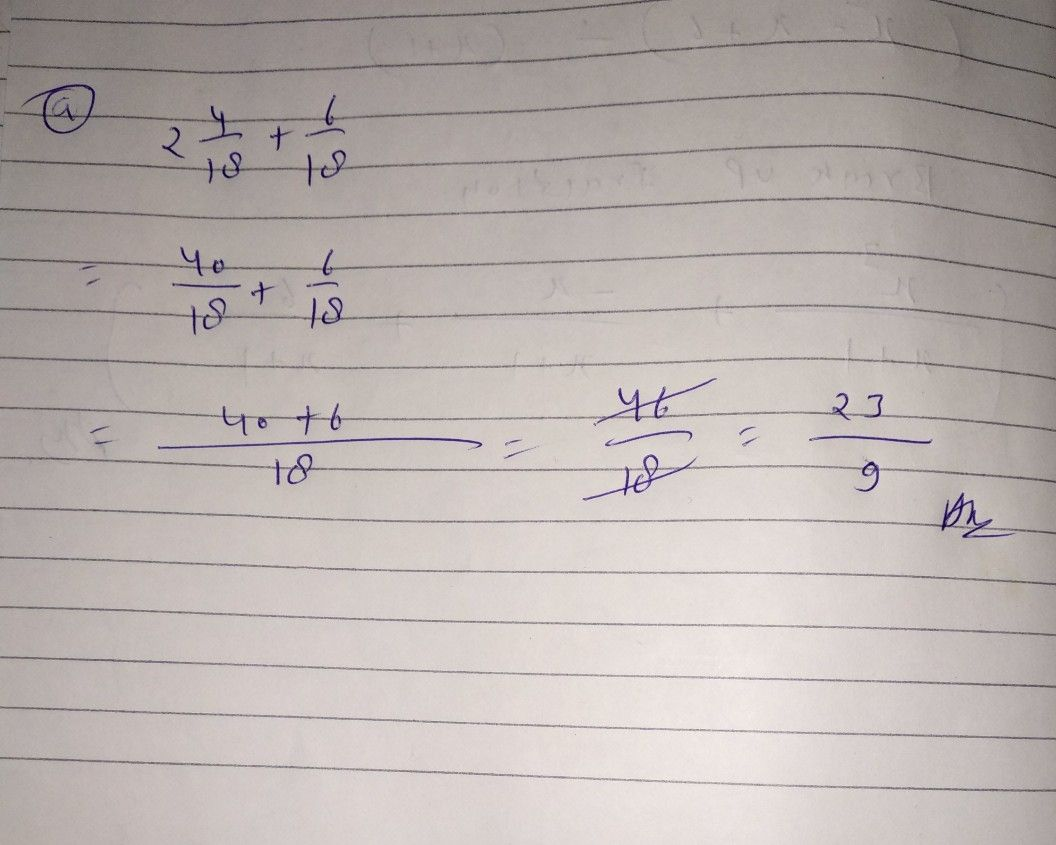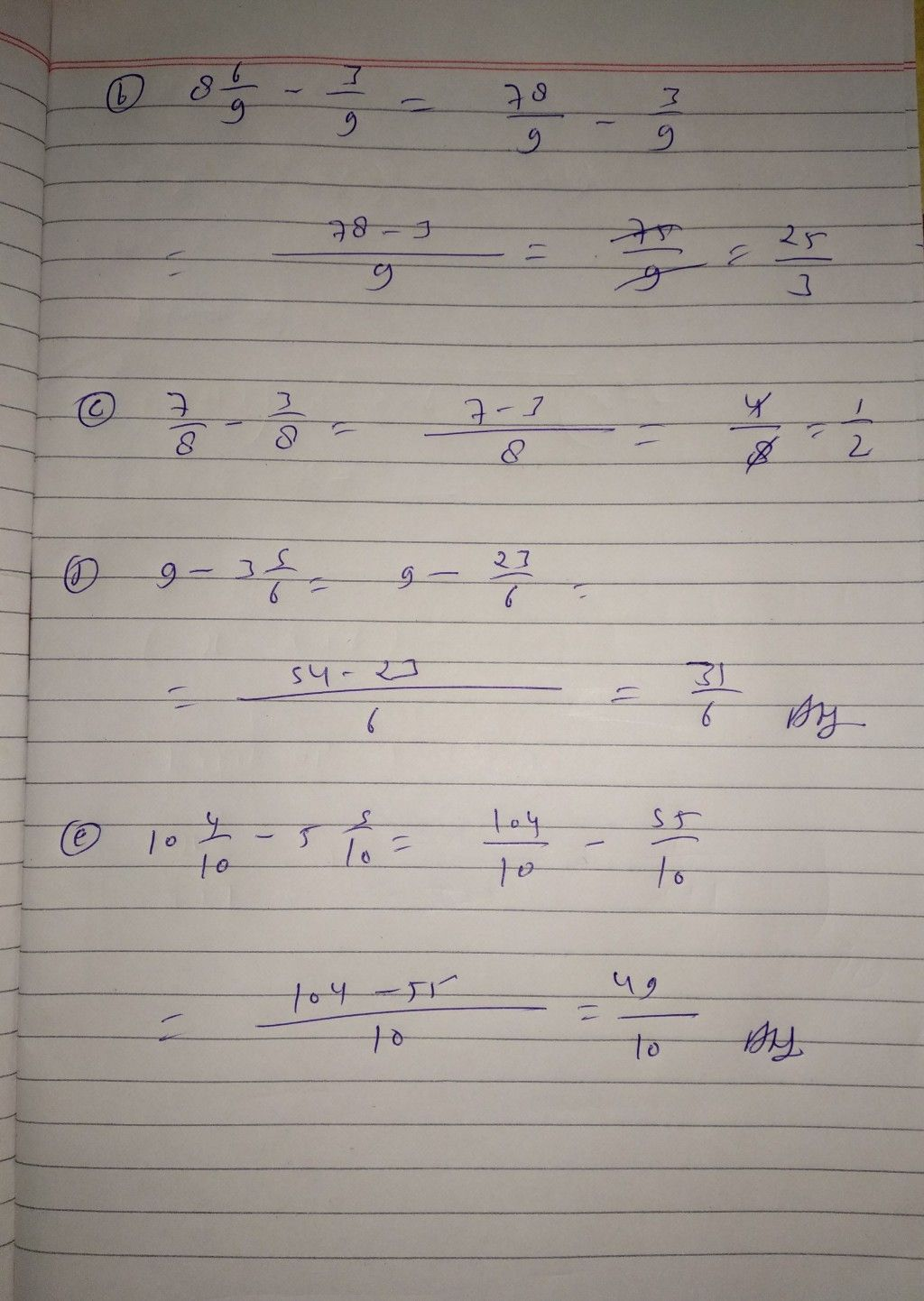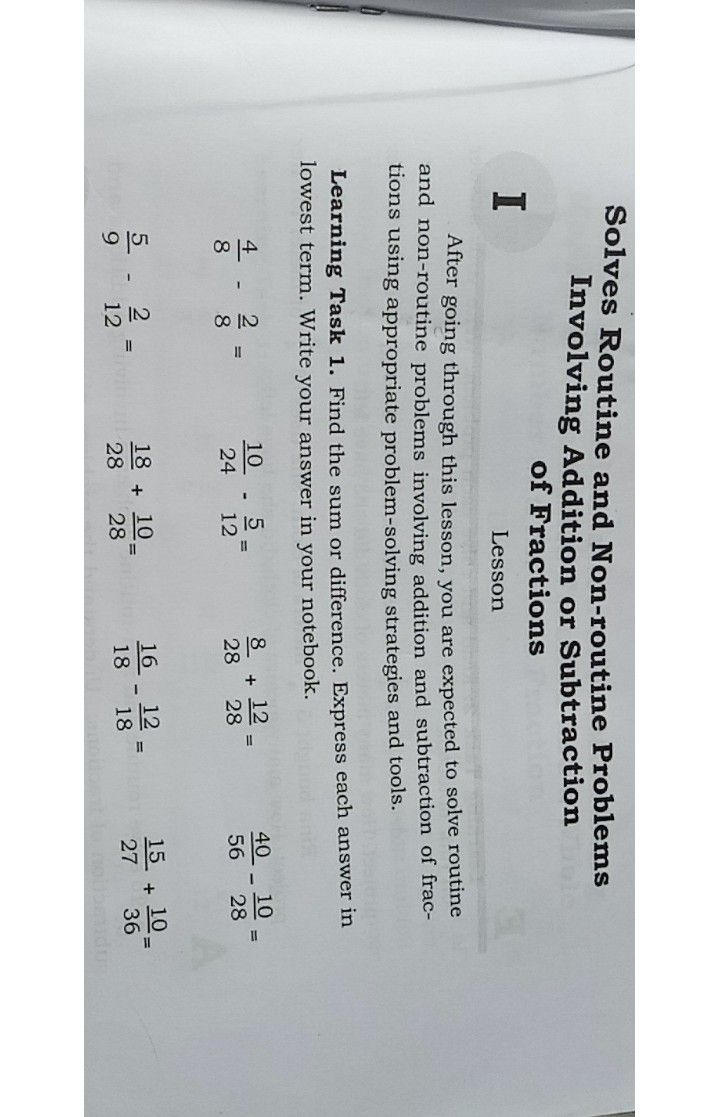Symbol
ProblemYou already know how to add similar fraction in lower grade. When fraction have common denominator, you just add the numerator and write the sum over the common denominator and write the lowest term. This time, learn more about addition and subtraction of fraction. In this lesson, you are expected to add and subtract fractions and mixed $\left(rac$ tion with and without regrouping. There is an activity below which will give you a review of this new lesson. Learning Task $1:$ Add or subtract the following fractions. Write you answer on your notebook. $G$ $2\dfrac {4} {18}+\dfrac {6} {18}$ $=$ $\square$ $c\right)$ $\dfrac {7} {8}$ $\dfrac {3} {8}=$ $8\dfrac {6} {9}$ $-\dfrac {3} {9}=$ d) $9-3\dfrac {5} {6}$ $=$ $\right)$ $10\dfrac {4} {10}-5\dfrac {5} {10}=$ D
1st-6th grade
Other
Search count: 105
SolutionQanda teacher - tyagiStudent
thank you
but i have an another question